## research papersBIOLOGICALCRYSTALLOGRAPHY
ISSN: 1399-0047
Volume 57| Part 10| October 2001| Pages 1367-1372

## Implementation of molecular replacement in AMoRe

aCNRS-GIF, LGV, 91198 Gif-sur-Yvette, France
*Correspondence e-mail: jorge.navaza@gv.cnrs-gif.fr

(Received 5 March 2001; accepted 12 June 2001)

An account is given of the molecular replacement method as implemented in the package AMoRe. The overall strategy of the method is presented and the main functions used in the package are described. The most important features of AMoRe are the quality of the fast rotation and translation functions and the facility of multiple inputs to translation and rigid-body refinement functions, which allow for a fast multiple exploration of crystal configurations with a high level of automation.

### 1. Introduction

The idea of molecular replacement is to build a tentative crystal structure using known molecular models similar to the actual molecules that constitute the crystal in order to start model building or refinement. The problem is to determine the positions of the models within the crystal cell. This is ultimately performed by comparing observed and calculated structure factors for selected positions of the independent molecules within the cell. In AMoRe, the comparison essentially involves the correlation coefficient in terms of amplitudes. This criterion was chosen in the light of the results available one decade ago, results that now may be considered as corresponding to easy or moderately difficult MR problems. At that time, an exhaustive positional search involving in general six variables per independent model using that simple but robust criterion could not be envisaged. Nowadays, a full six-dimensional search would also be too lengthy, although feasible. This explains, perhaps, the fact that the original ideas of Rossmann and Blow, i.e. the splitting of the search into two consecutive three-dimensional ones, are still found in filigree in most MR packages.

The main programs in AMoRe aim at selecting a certain number of positions, obtained through the exhaustive exploration of three-dimensional domains with fast functions, and computing the correlation coefficients associated with these positions. The idea is to assess many crystal configurations, as it is the contrast in the values of the criterion that gives one confidence in the solution. The fast functions, rotation functions and translation functions are either improved versions of already proposed ones or new ones. Accurate and fast algorithms are used throughout the package in order to save computing time. In particular, molecular scattering factors replace coordinates, which are used only once in the whole procedure.

The main stream in AMoRe is the set of values of the variables that specify the positions of the independent models within the crystal, from which structure factors and inputs to the fast functions are calculated. We will first define these variables and their relationship to the calculated structure factors. We will then describe the strategy for the selection of configurations.

### 2. Positional variables and crystal configurations

The position of the molecular model within the crystal is determined by the rotation R and the translation T that move the model from a reference initial position, specified by the atomic vectors {ro}, to the current position, specified by the atomic vectors {r},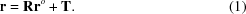The translation T is usually given in fractional coordinates (xyz) in the crystal cell. The rotation R is parameterized with the Euler angles (φ, θ, ψ) associated with an orthonormal frame (X, Y, Z). Several conventions exist for the names of angles and definitions of the axes involved in this parameterization. We will follow the convention by which (φ, θ, ψ) denotes a rotation of ψ about the Z axis, followed by a rotation of θ about the Y axis and finally a rotation of φ about the Z axis,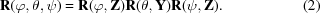The angles take values within the parallelepiped {0 ≤ φ < 360; 0 ≤ θ ≤ 180; 0 ≤ ψ < 360°}. For θ = 0 or 180°, only the combinations φ + ψ or φψ are independent, respectively.

The initial position of the model is usually chosen with its center of mass placed at the origin and its principal axes of inertia parallel to the orthonormal frame, as this leads to an efficient sampling of configurations. A good choice for the orthonormal frame is Z parallel to the highest crystal symmetry axis (nort = 0 in AMoRe). This choice restricts the orientational search to {0 ≤ φ < 36/n}, where n is the order of the rotational symmetry around Z.

Therefore, given the models' initial positions, the crystal unit-cell parameters, the space-group symmetry and the orientation of the orthonormal frame, a crystal configuration is uniquely determined by giving the positions of the independent molecular models within the unit cell, expressed in terms of the positional variables,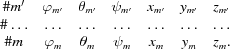The labels m′, …, m identify the molecules and the molecular models. Note that some of these models may coincide.

### 3. Structure-factor calculation

The calculated structure factors are conveniently written in terms of the individual molecular scattering factors fm(s), i.e. the Fourier transform of the electron density corresponding to the isolated molecule in its initial position. These molecular scattering factors are computed with the TABLING program, which translates the model coordinates so that the center of mass is at the origin and rotates the coordinates so that the model's principal axes of inertia are parallel to the model box. An electron density is then constructed and eventually transformed by Fast Fourier techniques. One feature of AMoRe is that the model may well be an electron density or an electron-microscopy reconstruction, as only the Fourier coefficients are used.

If Rm and Tm denote the rotation and translation that define the molecule's current position, Mg and tg the space-group transformation matrix and translation vector of the gth symmetry operation and H the coordinates of a crystal reciprocal vector, the contribution of molecule m to the calculated crystal structure factor is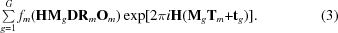D and Om are orthogonalizing and deorthogonalizing matrices. In fact, DRmOm is simply the rotation matrix Rm expressed in a mixed basis: it applies (from left to right) to reciprocal coordinates (Miller indices) in the crystal and produces reciprocal coordinates in the model box. If there are M independent molecules we have to add M terms like this. Assuming that the individual molecular scattering factors fm(s) have been set to a common scale, we have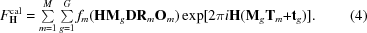### 4. Correlation coefficient

As stated in the introduction, the agreement criterion to assess crystal configurations is the (linear) correlation coefficient between observed and calculated amplitudes,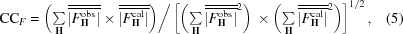where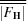denotes a centered' variable, e.g.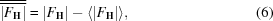and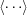means average over reflections. CCF takes values in the interval (1, −1).

### 5. Strategy

The overall strategy of MR as implemented in AMoRe is easily understood if we consider the correlation coefficient between intensities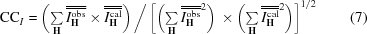as the target function for screening. The calculated total intensity is given by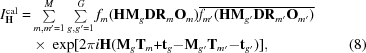where the overline means complex conjugate'. The positional variables entering into this expression are successively determined by using different approximations to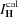and, accordingly, CCI. The protocol consists of three main steps.

 (i) Rotation search. For each of the M molecules, determine their possible orientations. The calculated intensities are approximated by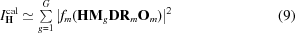and the highest peaks of CCI (as a function of Rm) are selected. This function is essentially the direct rotation function (Delano & Brünger, 1995). Even though CCI, withgiven by (9), cannot be calculated by standard fast techniques, available computing resources allow for a point-by-point evaluation of CCI at an average speed of ∼3 × 10−7 s per reflection and per symmetry operation on a 500 MHz Digital XP1000. (ii) One-body translation search. For each molecule and for each selected orientation, determine the possible translations. The calculated intensities are approximated by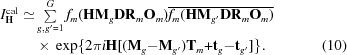CCI (as a function of Tm) is evaluated by FFT (Navaza & Vernoslova, 1995) within the Cheshire cell. The assessed (partial) crystal configurations are given by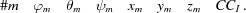The positions corresponding to the top one-body configurations are then refined; that with highest value of CCI is assumed to be correct. (iii) n-body translation search. When many independent molecules or molecular fragments have to be positioned, the contribution of already placed models dramatically increases the chances of success of the method. If M′ < M molecules are already positioned (say those with labels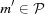), then for the top orientations of the remaining molecules (m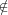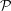), determine the possible translations. The calculated intensities are approximated by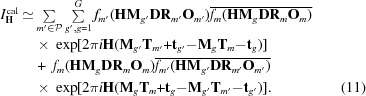CCI (as a function of Tm) is evaluated by FFT, now within the whole crystal cell. The assessed (partial) crystal configurations are now given by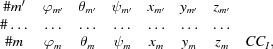where CCI corresponds to the whole (M′ + 1)-body configuration. The positions corresponding to the best configurations are then refined and that with highest value of CCI is assumed to be a correct one. (iv) Repeat the preceding step until M′ = M.

The actual protocol in AMoRe differs from the one above mainly in the rotational search. The ROTING program, based on the fast rotation function proposed by Crowther, is used to determine the possible orientations of the models (Crowther, 1972). Also, as previously stated, the crystal configurations are assessed with CCF instead of CCI. The translations of the oriented models (one-body and n-body searches) are determined with the TRAING program. Several translation functions have been incorporated, among which the one described in the above protocol, i.e. CCI as a function of Tm. The refinement of the positional variables is performed with the fast rigid-body refinement program FITING (Castellano et al., 1992). These fast functions will be described in the following section.

A situation where this protocol fails is often one in which a six-dimensional search fails too. As a rule, this corresponds to a poor quality of the search model or a small size of the search fragment with respect to the asymmetric unit content.

The fast structure-factor calculation algorithm (4), the performance of ROTING and the facility of multiple inputs to TRAING and FITING allow for a fast multiple exploration. A link between the input/output of the above programs allows for automation. In fact, three levels of automation may be distinguished.

 Level I: automation is here reduced to the possibility of multiple inputs to the main programs. Level II: inputs to the main programs are created by selecting and combining putative solutions. Level III: a protocol for the whole MR problem is created, starting from general purpose control parameters.

### 6. Description of the fast search programs

#### 6.1. The ROTING program

It is possible to determine the rotations R that superimpose a search molecule upon the homologous ones within the target crystal by calculating the overlap within a conveniently chosen region Ω of volume v of the observed Patterson function (the target function Pt) and a rotated version of the Patterson function corresponding to the isolated search molecule (the search function Ps),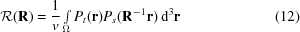(Rossmann & Blow, 1962).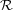should display a local maximum for the sought rotations. Note that when we rotate the search function Ps by R, its argument contains R−1.

It may be useful to compare rotation functions obtained under different conditions. For this, some kind of normalization is needed. In fact,is cast into the form of a correlation coefficient by dividing (12) by the norms of the truncated Patterson functions,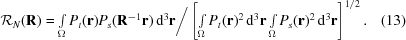The reciprocal-space formulation of (12) is obtained by replacing the Patterson functions by their Fourier summations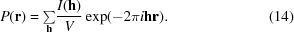Taking into account that I(−h) = I(h), we obtain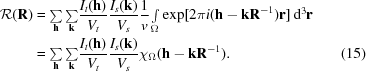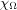is the Fourier transform of the function that takes the value 1 within Ω and 0 outside. In principle, the domain of integration could have any shape. However, in order to take full advantage of the properties of the rotation group, Ω is usually chosen as a spherical domain of radius b. Letting s = hkR−1 for short, we haveAlthough simple, the resulting expression for the rotation function has the disadvantage of containing entangled h, k and R contributions, which renders its computation time consuming if the whole domain of rotations has to be explored. The difficulty may be overcome by expanding the exponentials entering into (15) in spherical harmonics, Yl,m. Taking advantage of their transformation under rotations and using recurrence relationships between spherical Bessel functions jl, we obtain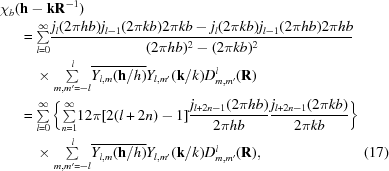where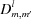are the matrices of the irreducible representations of the rotation group. The awkwardness of (17) is apparent rather than real.

 (i) The expression separates angular from crystal variables. It also separates target from search contributions. (ii) The equation is accurate, even when truncating the summations on l and n to reasonable values. The upper limit for l is of the order of the highest argument of the spherical Bessel functions,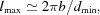where dmin is the resolution of the data. The upper limit for n depends on the current value of l,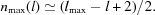(iii) When the rotations are parameterized in Euler angles (φ, θ, ψ), the matricestake the form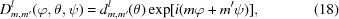which enables the computation offor each given value of θ by means of two-dimensional fast Fourier transforms.
This formulation is referred to as the fast rotation function (Crowther, 1972).

#### 6.2. Computing the fast rotation function

The calculations are organized as follows.

 (i) Given the search and the target diffraction data, compute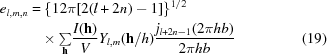and normalize (to compute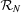instead of)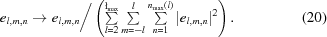Odd l terms disappear because the Friedel related reflections contribute with opposite signs,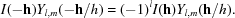Also, if the Patterson function has an n-fold rotation axis along Z, only the terms with m equal to a multiple of n survive. (ii) Given the el,m,n values, perform the sums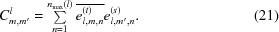(iii) For each θ value, calculate the reduced matrix elements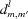and compute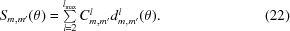Then evaluate the β section ofby FFT,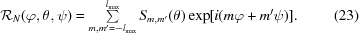The sampling in φ and ψ here is dictated by the standard FFT requirements.is used in AMoRe just to select a certain number of peaks. The output of ROTING contains, besides the values of, those of the correlation coefficients (CCF and CCI as in P1) for each of the selected orientations. CCF is more efficient, in general.

#### 6.3. The locked rotation function

The rotational NCS, determined with the help of the self-rotation function, may be used to enhance the signal-to-noise ratio of cross-rotation functions (Rossmann et al., 1972; Tong & Rossmann, 1990). If Sn, n = 1, …, N denotes the set of NCS rotations, including the identity, and R is a correct orientation of the cross rotation, then SnR must also correspond to a correct orientation. Here, we are assuming that the rotational NCS forms a group. Otherwise, either SnR or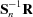, but not both, corresponds to another correct orientation. Therefore, a function may be defined, the locked cross rotation, whose values are the average of the values ofat orientations related by the NCS,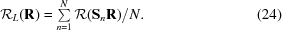By redefining the target function, it can be computed as an ordinary cross rotation. Indeed,L may be written in a form similar to (12),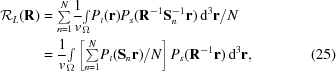with the target Patterson function substituted by the average over the NCS of the rotated target functions. The computation of (25) is particularly simple in the case of the fast rotation function. The substitution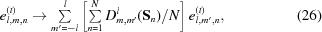where we replaced the sum over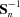by a sum over Sn, because of the rearrangement theorem of group theory, gives the required target coefficients.

#### 6.4. The TRAING program

The possible translations of an oriented model are selected in AMoRe by means of fast translation functions computed with the TRAING program. The output of this program contains, besides the values of the fast translation function, those of CCF, CCI and the R factor for each of the selected translations. Several fast translation functions may be calculated. If we write the Fourier coefficient of the oriented model, rotated by a given Rm and placed at T, as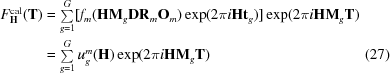(see equation 3) and the corresponding intensity as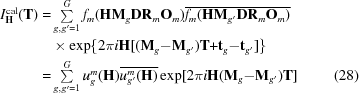(see equation 10), then the options are (same notation as in equations 6and 7)

• (i) centered overlap,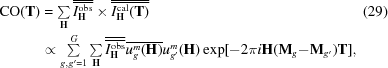• (ii) Harada–Lifchitz (Harada et al., 1981)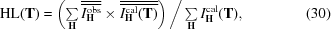• (iii) correlation coefficient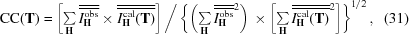• (iv) phased translation

 (a) without external' phases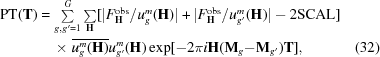(b) with external' phases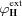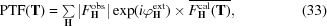• (v) n-body translation with fixed contribution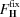(a) phased translation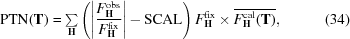(b) for all others, replace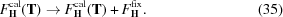SCAL is a scale factor to subtract the contribution of the phasing position. The complex exponentials in (29) to (32) depend on reciprocal vectors H(MgMg), which are in the Cheshire reciprocal cell (Hirshfeld, 1968).

#### 6.5. The FITING program

Although FITING is not a search program, we include it here as it is one of the main molecular-replacement programs. It performs rigid-body refinement by a fast technique first proposed by Huber & Schneider (1985). The quadratic misfit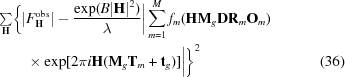is minimized with respect to the positional variables {Rm, Tm}, the overall scale factor λ and the overall temperature factor B.

### ReferencesBIOLOGICALCRYSTALLOGRAPHY